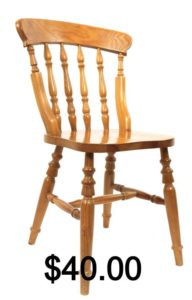## Why It Matters: Polynomial and Rational ExpressionsArrangement of US currency; money serves as a medium of financial exchange in economics.

## Why learn how to use polynomials and rational expressions?

In economics we learn that profit is the difference between revenue (money coming in) and costs (money going out).  Positive profit means that there is a net inflow of money, while negative profit means that money is being lost.

Suppose that you own a company that manufactures and sells furniture.  A particular chair sells for $40. If your company builds and sells 500 of these chairs in one month, then your monthly revenue would be$20,000. In general, if you manufacture and sell $x$ chairs, then the revenue $R$, in dollars, would be given by the formula:

$R=40x$

Now let’s talk about costs. Let’s say it costs $10,000 each month just to keep the factory open, regardless of how many chairs are produced. This amount might include the electric and gas bills as well as the monthly paychecks for each of your employees. These costs, which do not depend on the number of items produced, are called the overhead or fixed costs. Furthermore, suppose the materials cost$25 per chair. That means that if we produced $x$ chairs, then the total cost for materials would be $25x$. The costs which vary based on the number of items produced are called variable costs. Together, with the overhead, we can derive a total estimated cost formula.

$C=\left(overhead\right)+\left(variable\: costs\right)=10,000+25x$

Both formulas for revenue and cost are examples of polynomials. In fact both polynomials are what we call degree 1, or linear polynomials, because the variable $x$ only occurs with exponent 1.  What is the profit formula?  Simply subtract the two expressions  (Profit = Revenue – Costs), to find:

$P=R-C$

$P=\left(40x\right)-\left(10,000+25x\right)$

$P=40x-10,000-25x$

$P=15x-10,000$

Will your company make money if it manufactures and sells 500 chairs?  What about 800 chairs?

Now imagine that your small company has received more and more business over the past few months. You’re producing so many chairs that you had to hire new employees, purchase new trucks and even plan for a larger factory. Those costs are adding up!

After carefully analyzing monthly expenses, you estimate the additional costs (above and beyond the overhead and variable costs) to be modeled by a degree 2 (or quadratic) term, $0.001x^2$. Here are your new cost and profit formulas:

$C=10,000+15x+0.001x^2$

$P=40x-\left(10,000+25x+0.001x^2\right)=-0.001x^2+15x-10,000$

These new formulas are also polynomials, but they behave differently than theprevious formulas for large values of $x$.

Finally, let’s suppose you want to find out whether it is still cost-effective to produce your \$40 chairs in mass quantities. Perhaps the best way to find out is to determine the average profit when $x$ chairs are manufactured and sold. As always, an average is equal to the total amount ($P$, for profit in this case) divided by the number of items ($x$).

$\Large\frac{P}{x}=\frac{-0.001x^2+15x-10,000}{x}$

This time, the formula for average profit is no longer a polynomial, but a rational function, which is a ratio of two polynomials.

In this module you’ll learn how to classify and perform algebraic operations on polynomials and rational functions. We will check back in on our company’s profits at the end of the module.